Einstein's Theory of Relativity

## Classical Mechanics

by   Paul Marmet
Chapter Six
of the Perihelion of Mercury.
6.1 - Conditions Controlling the Geometrical Shape of an Orbit.
The advance of the perihelion of Mercury given in equation 5.46 was calculated using perturbations of individual parameters. This advance can also be illustrated using geometrical considerations. Newton stated the universal law of gravitation which predicts an exact quadratic gravitational field around a mass. Newton has shown that in the gravitational field around a central body, all masses move in elliptical orbits independently of the mass of the orbiting body. According to classical mechanics, the necessary condition to get an exact elliptical orbit is for the mass to move in a gravitational field whose intensity decreases exactly as the inverse of the square of the distance R from the central mass:6.1
There are several measurements showing that this quadratic decrease of the gravitational field is followed quite accurately in nature. At a distance RM(o.s.) from M(S)(o.s.), the field is given by:6.2
where G(o.s.) is the number of outer space units of the gravitational constant and M(S)(o.s.) is the number of outer space units of the solar mass. Equation 6.2 implies that the Sun generates an exact quadratic gravitational field (in outer space units) in which Mercury is submerged.
Although the inverse quadratic law is generally accepted, a very slight deviation of that law was first suggested by Aseph Hall in 1894 . Since we have seen that the mass of a body changes when it is moved into a gravitational potential, we can show that such a slight change of mass leads to an effect equivalent to the slight change of the quadratic function suggested by Hall.
Classical mechanics shows that a massive body travels in an elliptical orbit when the force F rather than the field between the central mass and the orbiting mass decreases as the square of the distance. Let us consider Newton's equation (written in a correct way, contrary to equation 5.1):6.3
Since the mass of Mercury changes with its distance from the Sun, it is incorrect to believe that the force between the Sun and Mercury still follows an inverse quadratic function of that distance. Even if the gravitational field around a central mass decreases exactly as the square of the distance, the total force between Mercury and the Sun does not decrease at the same rate as the field. The trajectory of a planet whose mass decreases when it gets deeper in a gravitational field corresponds exactly to the problem of a non quadratic force around a central mass. Using classical mechanics we can calculate the new geometrical shape of the orbit when the force (not the field) between the Sun and Mercury is non quadratic.
However, when we consider the proper parameters of the observer moving to different distances from the Sun, the gravitational field (defined as the force divided by the proper mass) calculated from equation 5.9 is not quadratic for the observer traveling between different locations from the Sun. Consequently, using the parameters existing where Mercury interacts with the gravitational field leads to an apparent non quadratic field (since the proper mass of Mercury is constant for a Mercury observer).
Using either the non quadratic force as seen by an outer space observer that takes into account the change of mass of Mercury or the apparent non quadratic force given by equation 5.9 (with constant proper mass) leads to a similar advance of the perihelion of Mercury. However, these calculations are incomplete because other fundamental phenomena, like the change of mass as a function of the velocity of Mercury on its orbit, are not taken into account. Changes of length and clock rate due to Mercury's velocity and gravitational potential should also be taken into account.
Since we have already calculated the total precession in equation 5.46, we will limit our demonstration here to the change of one parameter using only the change of mass of Mercury as a function of its distance from the Sun. We will use only the perturbation of this parameter and show that it is one of the contributions to the geometrical precession of the ellipse which can be illustrated in a classical experiment that can be done in a laboratory using a simple apparatus.

6.2 - The Change of Mass of Mercury.
Let us consider the change of force on Mercury due to its change of mass as a function of its distance from the Sun. Equation 4.25 shows how the absolute mass of a kilogram decreases when getting closer to the Sun. Consequently, the total mass of Mercury decreases by the same ratio. From equations 4.39, 4.40 and 4.41, the mass of Mercury (in outer space units) follows the relationship:6.4
Equations 6.4 and 4.25 give:6.5
or: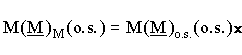6.6
Using equation 6.6 in 6.3 gives a force equal to:6.7
which is equal to: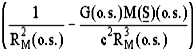6.8
Let us define:6.9
Equation 6.8 becomes: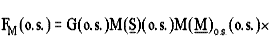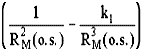6.1
Equation 6.10 shows that the gravitational force is the difference between a quadratic and a cubic function. It is known that in a quadratic field, an elliptical orbit with a small eccentricity (first order expansion) follows the equation r = a(1 + ecosq) (a is the semi-major axis and e is the eccentricity). This equation implies two components: a tangential component of constant radius a and a radial component of amplitude aecosq. Since Kepler's third law predicts the same period (first order) for orbits having the same average radius with or without eccentricity, both the tangential and the radial components lead to the same period in a quadratic field.
However, in the case of a non quadratic field (cubic term in equation 6.10), the period of oscillation of the radial component becomes longer than the period of the circular tangential component. Of course, a circular component does not 'feel' the field gradient. Because the cubic radial component of oscillation has a longer period, there is a continual shift of phase between the periods of the tangential and of the radial components.
Consequently, the cubic term in equation 6.10 which does not follow Kepler's quadratic gradient of force, is responsible for the precession of the ellipse because the radial component, having a longer period, becomes out of phase with the circular component. It is the difference of period between the tangential and the radial components of motion that produces the precession of the ellipse. We also notice that it is the radial component of oscillation which is most affected by the change of parameters resulting from mass-energy conservation.
Let us examine the bracket on the right hand side of equation 6.10. Using a series expansion, we can show that it is mathematically equivalent to a simple exponential form given by:6.11
in which the exact value of e is:6.12
A very good approximation to the first order (with n = 1) gives:6.13
Combining equations 6.10 and 6.11 gives:6.14
where e is always positive. Equation 6.14 shows that, because of the decrease of mass due to mass-energy conservation, the force F between Mercury and the Sun no longer decreases exactly as the square of the distance. The change of mass of Mercury as a function of its distance from the Sun is responsible for the change of power of RM from 2 to 2+e. Therefore even if the gravitational field affecting Mercury decreases exactly as the inverse of the square of the distance as written in equation 6.2 (as in a perfect Newtonian field), the gravitational force is not Newtonian as shown in equation 6.14. Let us reconsider now the trajectory of bodies submitted to a force decreasing with a function which is slightly different from 1/R2.

6.3 - Orbital Shapes and Gravitational Force Gradients.
We have calculated in equation 6.10 the force on Mercury as a function of the distance RM. The corresponding gravitational potential VM(o.s.) is obtained by the integral of equation 6.10. This gives:6.15
The orbit followed by a mass submitted to the potential described by equation 6.15 has already been calculated [2, 3]. Using temporarily Goldstein's notation , the solution of equation 6.15 is a precessing ellipse with a velocity of precession equal to: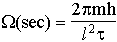6.16
where W(sec) is in radians per second of time. Transforming Goldstein's notation into ours, we have m = M(M)o.s.(o.s.) and h = (G(o.s.)M(S)(o.s.)M(M)o.s.(o.s.)k1)/2. t is the period of translation of Mercury around the Sun. The angular momentum l in equation 6.16 is:6.17
where dq/dt is the angular velocity. Therefore, from equation 6.9 and the definitions above, we have: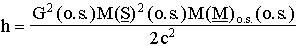6.18
From equations 6.16, 6.17 and 6.18, we have: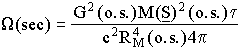6.19
Let us transform the precession W(sec) given in radians per second for radians per circumference W(circ). We obtain:6.2
By definition, the period t equals:6.21
Equation 6.21 in 6.20 gives:6.22
Newton's law shows that the force of gravity FG is equal to the centrifugal force FC in a circular orbit (the eccentricity has not yet been taken into account). We have the fundamental equations:6.23
Equation 6.23 gives:6.24
Equations 6.24 and 6.22 give:6.25
Equation 6.25 gives the velocity of precession of an ellipse for the case of a perfect quadratic field in which the orbiting mass changes with its position in the gravitational potential, due to mass-energy conservation.

6.4 - Identity of Mathematical Forms.
We find that the advance of the perihelion of Mercury obtained with the perturbation method used by Einstein and by us in equation 5.46, has the same mathematical form as equation 6.25 which clearly corresponds to the precession of an elliptical orbit. There are two obvious differences. Since we have not taken into account the eccentricity of the orbit, the term 1-e2 is naturally missing in equation 6.25 as explained in section 5.10. Other similar parameters are ignored here since we do not take into account the perturbations explained in section 6.1. If we take into account these perturbations, other similar terms will be added and the full precession will be found as obtained in chapter five. The aim of the present demonstration is only to illustrate the reality of the classical precession of the ellipse in the case of a non quadratic force.

6.5 - Illustration of Trajectories in Potential Wells.
When the force on a planet moving around the Sun decreases as the square of its distance from the Sun, it travels on a perfect ellipse. However, due to mass-energy conservation, the exact intensity of the force does not decrease as the square of the distance. As seen in equation 6.14 the force follows the relationship:6.26
The trajectory of a particle submitted to equation 6.26 is an ellipse as illustrated on figures 6.1 and 6.2. In figure 6.1, a smooth conic surface is built (in the Earth gravitational field) in such a way that the height above the ground increases as the negative of the inverse of the square of the distance from the central axis. This corresponds to e = 0 in equation 6.26. In this case, the potential energy of a ball sliding (without friction) on the surface increases according to the inverse quadratic function from the center. If we throw a ball on the surface, we can get a circular orbit at various distances from the center. Using a different initial angular momentum, one can observe a stationary elliptical orbit as drawn on figure 6.1.Figure 6.1
Demonstration of a mass moving in an elliptical orbit in a quadratic potential well changing as 1/r2.

However, if the shape of the cone is different (see figure 6.2) so that the potential increases more rapidly than the inverse square of the distance (corresponding to equation 6.26 with e ¹ 0), after throwing a ball, we see that the axis of the elliptical orbit precesses just as observed for Mercury in its orbit around the Sun. The cause of that classical precession on that apparatus is (in part) the same as the cause of the precession of 43 arcsec per century of Mercury. Of course, this demonstration assumes that the friction and the rotation of the ball are negligible.Figure 6.2
Demonstration of the precessing orbit of a mass moving in a potential well changing as 1/r(2+e).

This shows that the advance of the perihelion of Mercury is not caused by space or time distortion. It is simply a beautiful demonstration of classical mechanics that predicts precessing orbits giving the shape of a rosette.

6.6 - Validity of the Classical Model.
We have found above that there is a perfect mathematical agreement between the result calculated in equation 5.46 and the result predicted using Einstein's mathematics. Moreover, those results are in perfect agreement with the observations of the advance of the perihelion of Mercury.
In order to arrive to his equation, Einstein, needed several new hypotheses called Einstein's relativity principles. Let us compare the hypotheses used by Einstein with the ones used in this book to find the Lorentz transformations and the equation for the advance of the perihelion of Mercury. This comparison is important if we wish to apply Occam's razor which gives a preference to the theory that requires the minimum number of hypotheses. Einstein's theory requires many new hypotheses, for example:
1) the reciprocity principle which is not compatible with mass-energy conservation as showed in section 3.9;
2) the hypothesis that the acceleration produced by a change of velocity is undistinguishable from the acceleration due to gravity (see chapter ten);
3) the non conservation of mass-energy in general relativity.
Einstein then arrived at the consequences that space and time can be distorted, contracted and dilated. In fact, Einstein's model not only requires new physical hypotheses, it also requires "new logic" which is not compatible with the natural understanding of nature. Classical logic can no longer be applied in relativity. In this book, we use the Bohr model of the atom which is so familiar everywhere in physics. We also find that using proper values, the physical relationships are valid in all frames as in Einstein's relativity. At the same time, a rational explanation is given. No time nor space distortion is required and the new interpretation is compatible with classical logic. There is certainly an extremely strong preference in favor of this new model when we apply Occam's razor.

Important Note:
After the writing of that book, a complete detailed description of the advance of the perihelion of Mercury entitled:
"A Detailed Classical Description of the Advance of the Perihelion of Mercury".

This new paper appears: At this location

6.7 - References.
 A. Hall, A Suggestion in the Theory of Mercury, Astr. J. 14, 49-51, 1894.
 H. Goldstein, Classical Physics, Addison-Wesley, Reading, Mass., second Edition, p. 123, 1980.
 E. T. Whittaker, A Treatise on the Analytical Dynamics of Particles and Rigid Bodies, Cambridge University Press, Fourth Edition, Chapter 4, 1937. (also Dover, New York, 1944).

6.8 - Symbols and Variables.

 FM(o.s.) number of outer space newtons for the gravitational force on Mercury G(o.s.) number of outer space units for the gravitational constant kgframe mass of the local kilogram in absolute units M(M)M(o.s.) number of outer space kilograms for Mercury at Mercury location M(M)o.s.(o.s.) number of outer space kilograms for Mercury in outer space m(M)M[o.s.] mass of Mercury in outer space units at Mercury location m(M)o.s.[o.s.] mass of Mercury in outer space units in outer space M(S)(o.s.) number of outer space units for the mass of the Sun RM(o.s.) number of outer space units for the distance of Mercury from the Sun VM(o.s.) number of outer space units for the gravitational potential on Mercury
<><><><><><><><><><><><>
Chapter5     Contents      Chapter 7